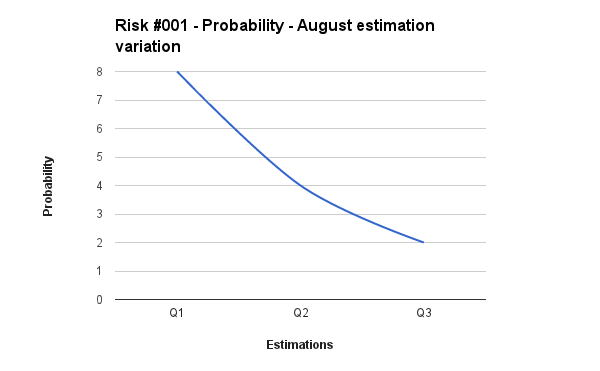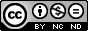## Monday, November 12, 2012

### A structured approach to qualitative risk analysis

Have you ever undergone the terrible experience of needing a plumber on Christmas day?
If your answer is "yes", you will have learned the hard way that the impact of a random event is a function of time. The same can be said about the probability.

So, when it comes the time for qualitative risk analysis, is rather a simplistic approach, assign constant values for risks' probability and impact, not considering the time dependency that these variables have.

Normally, qualitative risk analysis is a prelude to the quantitative one, in which a thorough risks review is carried out with appropriate statistical tools and methods.

Nevertheless, a structured approach for risks qualitative analysis could be helpful for projects which
• Are too small to justify both qualitative and quantitative risk analysis.
• Have so many risks, that performing a complete quantitative analysis could be too much time and resource consuming.

So, my suggestion is that every time a qualitative analysis is performed
• Assess probability and impact values for all the project risks, for the entire project life cycle. Do not assign constant values that are meaningful just for the here and now. Try to forecast future values and reasses past ones, expressing them as a function of time, using all the information you can get.
• Collect, analyze, and potentially correct all your previous estimations.

Please, take a look at the next two images.
The qualitative risk analysis of Risk #001 has been performed as a function of time and covers the expected project life cycle.

Figure 1 depicts the qualitative value assigned to the occurrence probability of the risk; figure 2 represents the qualitative value assigned to its impact.
Both values  are provided for the entire project time horizon and depicted as functions of time.Figure 1: Probability as a function of time.Figure 2: Impact as a function of time.

As we can see, a quantitative analysis of the current risk could be quite safely avoided until May.
So, indications to perform quantitative risk analysis on risk #001 in Q2 can be added to the risk management plan.
During project execution, if new evaluations would contradict the present data, risk #001 could immediately undergo the analysis process.

In figure 3 and figure 4 is shown the qualitative risk analysis temporal evolution for risk #001.
Every 3 months the qualitative risk analysis results have been questioned in the light of new information, obtained during project execution, and the assessments of probability and impact corrected.

The probability value has been set to zero for past months since the risk didn't occur.

The risk's impact value on the project has been instead reassessed also for past months.
The reassessment process is useful for following reviews of the qualitative risk analysis effectiveness.Figure 3: Probability as a function of time. Qualitative analysis performed each 3 months.Figure 4: Impact a function of time. Qualitative analysis performed each 3 months.

In figure 5 it can be seen an estimation of the accuracy of the qualitative risk analysis performed on risk #001.
The horizontal lines represent the risk impact mean values of all the evaluations performed during the project life cycle (actual values can be seen in Figure 4) while the vertical lines depict the standard deviation values (mean +/- sigma).Figure 5: Impact. Means and standard deviations for each estimation as a function of time.

The showed graphical representation can be very useful during project closure and lessons learned collection. Figure 5 shows how well (or how bad) we have performed qualitative risk analysis.
The presented approach helps project managers to understand how to refine estimations, and the degree of uncertainty it can be expected for similar projects.

Figure 6 and figure 7 depict the temporal evolution for the qualitative values of probability and impact for risk #001 (actual values can be seen in figure 3 and 4).Figure 6: Probability as a function of time for a single month estimation. Month is August. See also Figure 3.Figure 7: Impact as a function of time for a single month estimation. Month is September. See also Figure 4.

It can be seen that for the selected months, the probability assessed value decays with a quadratic law while the impact value changes linearly.
Mathematical models and polynomial fitting techniques can be used to discover hidden patterns in the data, and the velocity of convergence of an estimation to an unbiased value. Results could also be correlated with previously conducted analysis and grouped by risks area.

The proposed approach could be useful in aiding project managers to refine their estimations for future projects, it could display the natural tendency to overestimate or underestimate particular categories of risk in particular moments of the project life cycle, and can be used to debias estimations, conducting to more reliable forecasts.

The bottom line is that having qualitative evaluations for each project risk in the form of time function can be helpful for

• Determine when a particular risk deserves a quantitative analysis, plan the required actions and allocates the required resources well before the task can become critical.
• Select a proper statistical probability distribution to be used in quantitative analysis and in statistical simulations. For example it could be estimated fitting a model over the curves presented in the first 2 figures.
• Allocate contingencies in a more effective way.
• Get debiased estimations and more reliable forecasts.
• Assess the qualitative risk analysis effectiveness and correctness.
• Estimate the natural tendency of a particular project manager in overestimating or overestimating particular categories of risk in particular moments of the project life cycle.Quest' opera è distribuita con licenza Creative Commons Attribuzione - Non commerciale - Non opere derivate 3.0 Unported.

1.Your observation that the estimates vary over time is very interesting. It shows that one must think about when to re-examine risks. Some project managers whom I have only tracked the status of risks and the progress of risk mitigation measures. They never checked on changes to qualitative risk assessments.

I think one might take another step. Instead of reassessing risks on a milestone or calendar schedule (such as quarterly), one might ask, "When could we identify the warning signs that this risk will become an issue? When will be the latest date on which we can deal with this risk with low impact to the project?" One could then schedule the reviews at more optimal times.

1.Thank you Richard,
an adaptive risks review schedule is indeed very interesting.
What do you think should be the triggers to be identified or the indicators that should be checked ?